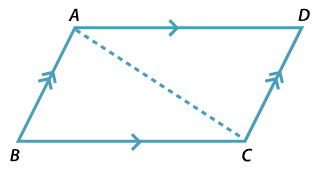### Using congruence to establish properties of parallelograms

#### Theorem

The opposite sides of a parallelogram are equal.

##### Proof$$ABCD$$ is a parallelogram. To prove that $$AB = CD \ \text{and} \ AD = BC$$, join
the diagonal $$AC$$ in the triangles $$ABC \ \text{and} \ CDA$$.

 $$\angle BAC$$ = $$\angle DCA$$ (alternate angles, $$AB \| DC$$) $$\angle BCA$$ = $$\angle DAC$$ (alternate angles, $$AD \| BC$$) $$AC$$ = $$CA$$ (common) So $$\triangle ABC \equiv \triangle CDA$$ (AAS) Hence AB = CD and BC = AD (matching sides of congruent triangles).